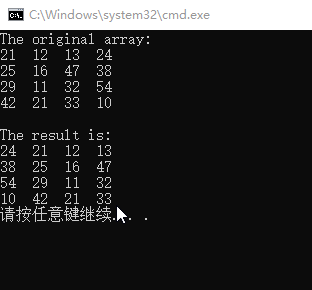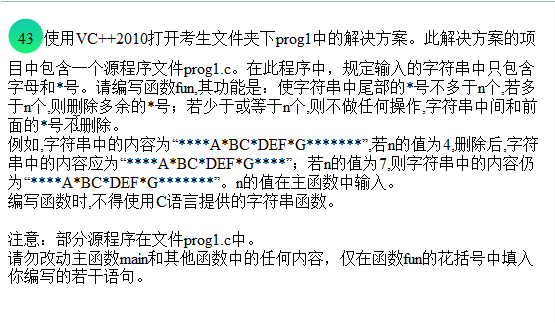# C语言二级真题

## 问题1：

…依次递推void fun(int  (*t)[N])
{  int  i, j, x;
/**********found**********/
for(i=0; i<N; i++)
{
/**********found**********/
x=t[i][N-1] ;
for(j=N-1; j>=1; j--)
t[i][j]=t[i][j-1];
/**********found**********/
t[i]=x;//最后一行移到第一行
}
}


## 问题2：void  fun( char *a,int  n )
{
char *p = a;
while (*p != '\0')
p++;
p--;//往前移一个位置
while (*p == '*')
p--;//定住最后一个字母 下一个就是*号
while (a < p)
a++;
int count = 0;
while (*a != '\0'&&count <= n)
{
a++;
count++;
}
*a = '\0';//字符串结束标志

}©️2019 CSDN 皮肤主题: 大白 设计师: CSDN官方博客